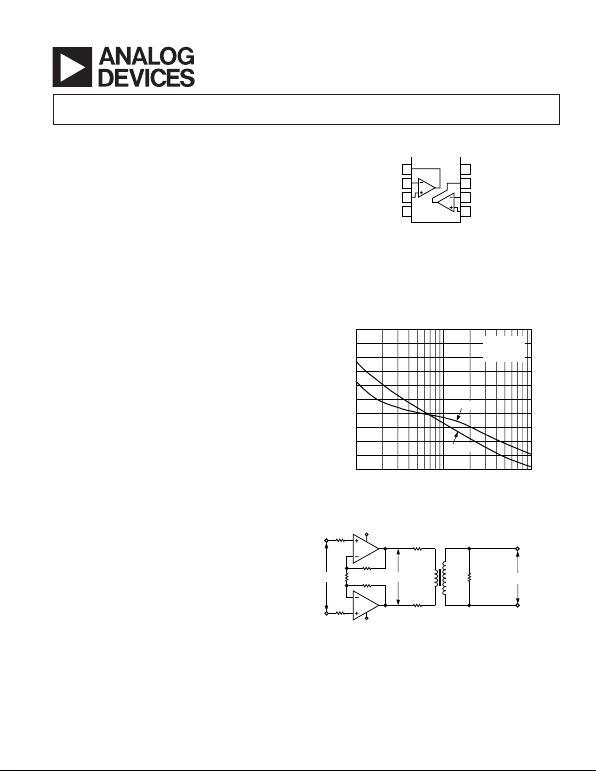REV. B
Information furnished by Analog Devices is believed to be accurate and
reliable. However, no responsibility is assumed by Analog Devices for its
use, nor for any infringements of patents or other rights of third parties that
may result from its use. No license is granted by implication or otherwise
under any patent or patent rights of Analog Devices. Trademarks and
registered trademarks are the property of their respective owners.
One Technology Way, P.O. Box 9106, Norwood, MA 02062-9106, U.S.A.
Tel: 781/329-4700 www.analog.com
*
Dual 350 MHz
Low Power Amplifier
*Protected under U.S. Patent Number 5,537,079.
FEATURES
Low Power
1.7 mA/Amplifier Supply Current
Fully Specified for 5 V and +5 V Supplies
High Output Current, 125 mA
High Speed
350 MHz, –3 dB Bandwidth (G = +1)
150 MHz, –3 dB Bandwidth (G = +2)
2,250 V/s Slew Rate
20 ns Settling Time to 0.1%
Low Distortion
–72 dBc Worst Harmonic @ 500 kHz, RL = 100
–66 dBc Worst Harmonic @ 5 MHz, RL = 1 k
Good Video Specifications (RL = 1 k, G = +2)
0.02% Differential Gain Error
0.06 Differential Phase Error
Gain Flatness 0.1 dB to 40 MHz
60 ns Overdrive Recovery
Low Offset Voltage, 1.5 mV
Low Voltage Noise, 2.5 nV/Hz
APPLICATIONS
XDSL, HDSL Line Drivers
Professional Cameras
CCD Imaging Systems
Ultrasound Equipment
Digital Cameras
FUNCTIONAL BLOCK DIAGRAM
8
7
6
5
1
2
3
4
OUT1
–IN1
+IN1
+V
S
OUT2
–IN2
+IN2–V
S
PRODUCT DESCRIPTION
The AD8012 is a dual, low power, current feedback amplifier
capable of providing 350 MHz bandwidth while using only
1.7 mA per amplifier. It is intended for use in high frequency,
wide dynamic range systems where low distortion and high
speed are essential and low power is critical.
With only 1.7 mA of supply current, the AD8012 also offers
exceptional ac specifications such as 20 ns settling time and
2,250 V/µs slew rate. The video specifications are 0.02% differ-
ential gain and 0.06 degree differential phase, excellent for such
a low power amplifier. In addition, the AD8012 has a low offset
of 1.5 mV.
The AD8012 is well suited for any application that requires high
performance with minimal power.
The product is available in standard 8-lead SOIC or MSOP
packages and operates over the industrial temperature range
40°C to +85°C.
R
L
–40
–9010 1k100
DISTORTION – dBc
–70
–80
–60
–50
G = +2
V
OUT
= 2V p-p
R
F
= 750
THIRD
SECOND
Figure 1. Distortion vs. Load Resistance, V
S
=
±
5V,
Frequency = 500 kHz
AMP 1
V
IN
V
REF
R2
R1
R
L
= 100
OR
135
V
OUT
Np:Ns
TRANSFORMER
LINE
POWER
IN dB
+V
S
+
–V
S
+
Figure 2. Differential Drive Circuit for XDSL Applications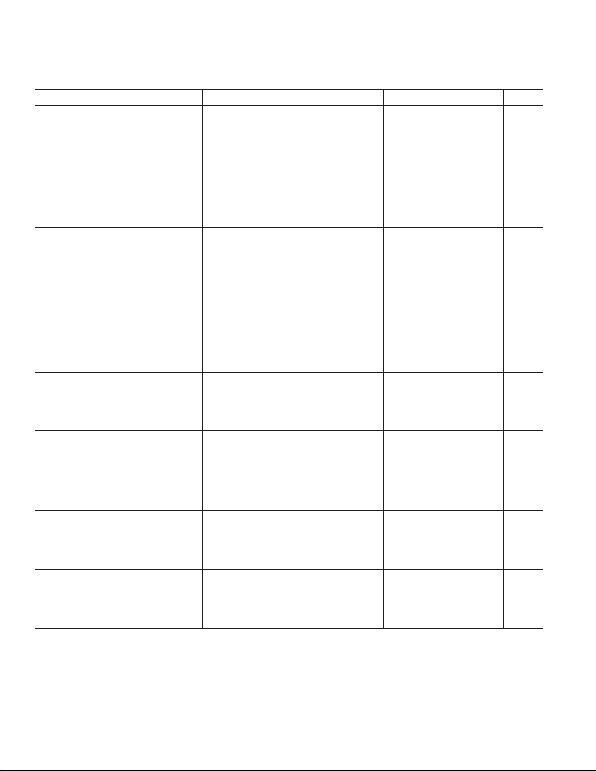REV. B–2–
Parameter Conditions Min Typ Max Unit
DYNAMIC PERFORMANCE
3 dB Small Signal Bandwidth G = +1, V
OUT
< 0.4 V p-p, R
L
= 1 k270 350 MHz
G=+2, V
OUT
< 0.4 V p-p, R
L
= 1 k95 150 MHz
G=+2, V
OUT
< 0.4 V p-p, R
L
= 100 90 MHz
0.1 dB Bandwidth V
OUT
< 0.4 V p-p, R
L
= 1 k/100 40/23 MHz
Large Signal Bandwidth V
OUT
= 4 V p-p 75 MHz
Slew Rate V
OUT
= 4 V p-p 2,250 V/µs
Rise and Fall Time V
OUT
= 2 V p-p 3 ns
Settling Time 0.1%, V
OUT
= 2 V p-p 20 ns
0.02%, V
OUT
= 2 V p-p 35 ns
Overdrive Recovery 2 Overdrive 60 ns
NOISE/HARMONIC PERFORMANCE
Distortion V
OUT
= 2 V p-p, G = +2
Second Harmonic 500 kHz, R
L
= 1 k/100 89/73 dBc
5 MHz, R
L
= 1 k/100 78/62 dBc
Third Harmonic 500 kHz, R
L
= 1 k/100 84/72 dBc
5 MHz, R
L
= 1 k/100 66/52 dBc
Output IP3 500 kHz, f = 10 kHz, R
L
= 1 k/100 30/40 dBm
IMD 500 kHz, f = 10 kHz, R
L
= 1 k/100 79/77 dBc
Crosstalk 5 MHz, R
L
= 100 70 dB
Input Voltage Noise f = 10 kHz 2.5 nV/Hz
Input Current Noise f = 10 kHz, +Input, Input 15 pA/Hz
Differential Gain f = 3.58 MHz, R
L
= 150 /1 k, G = +2 0.02/0.02 %
Differential Phase f = 3.58 MHz, R
L
= 150 /1 k, G = +2 0.3/0.06 Degrees
DC PERFORMANCE
Input Offset Voltage ±1.5 ±4mV
T
MIN
T
MAX
±5mV
Open-Loop Transimpedance V
OUT
= ±2 V, R
L
= 100 240 500 k
T
MIN
T
MAX
200 k
INPUT CHARACTERISTICS
Input Resistance +Input 450 k
Input Capacitance +Input 2.3 pF
Input Bias Current +Input, Input ±3±12 µA
+Input, Input, T
MIN
T
MAX
±15 µA
Common-Mode Rejection Ratio V
CM
= ±2.5 V 56 60 dB
Input Common-Mode Voltage Range ±3.8 ±4.1 V
OUTPUT CHARACTERISTICS
Output Resistance G = +2 0.1
Output Voltage Swing ±3.85 ±4V
Output Current T
MIN
T
MAX
70 125 mA
Short-Circuit Current 500 mA
POWER SUPPLY
Supply Current/Amp 1.7 1.8 mA
T
MIN
T
MAX
1.9 mA
Operating Range Dual Supply ±1.5 ±6.0 V
Power Supply Rejection Ratio 58 60 dB
Specifications subject to change without notice.
DUAL SUPPLY
(@ TA = 25C, VS = 5V, G = +2, RL = 100 , RF = RG = 750 , unless otherwise noted.)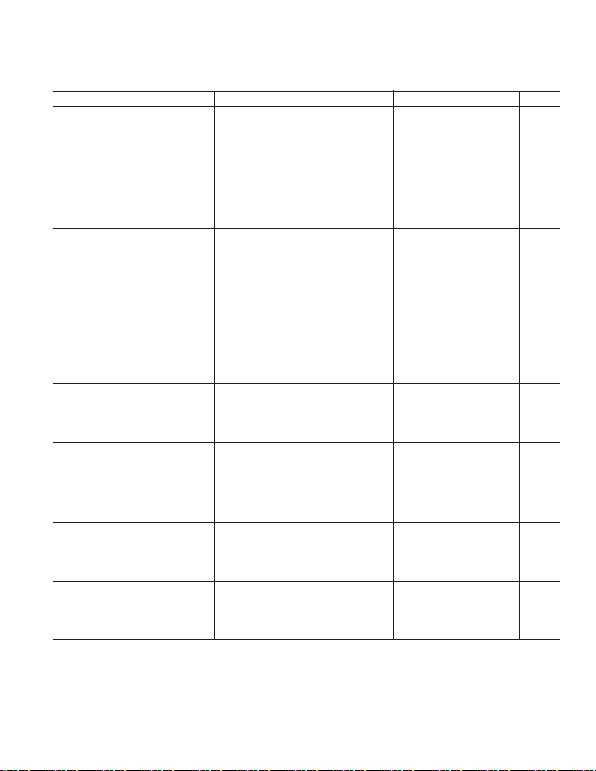REV. B
–3–
SINGLE SUPPLY
Parameter Conditions Min Typ Max Unit
DYNAMIC PERFORMANCE
3 dB Small Signal Bandwidth G = +1, V
OUT
< 0.4 V p-p, R
L
= 1 k220 300 MHz
G=+2, V
OUT
< 0.4 V p-p, R
L
= 1 k90 140 MHz
G=+2, V
OUT
< 0.4 V p-p, R
L
= 100 85 MHz
0.1 dB Bandwidth V
OUT
< 0.4 V p-p, R
L
= 1 k/100 43/24 MHz
Large Signal Bandwidth V
OUT
= 2 V p-p 60 MHz
Slew Rate V
OUT
= 3 V p-p 1,200 V/µs
Rise and Fall Time V
OUT
= 2 V p-p 2 ns
Settling Time 0.1%, V
OUT
= 2 V p-p 25 ns
0.02%, V
OUT
= 2 V p-p 40 ns
Overdrive Recovery 2 Overdrive 60 ns
NOISE/HARMONIC PERFORMANCE
Distortion V
OUT
= 2 V p-p, G = +2
Second Harmonic 500 kHz, R
L
= 1 k/100 87/71 dBc
5 MHz, R
L
= 1 k/100 77/61 dBc
Third Harmonic 500 kHz, R
L
= 1 k/100 89/72 dBc
5 MHz, R
L
= 1 k/100 78/52 dBc
Output IP3 500 kHz, R
L
= 1 k/100 30/40 dBm
IMD 500 kHz, R
L
= 1 k/100 77/80 dBc
Crosstalk 5 MHz, R
L
= 100 70 dB
Input Voltage Noise f = 10 kHz 2.5 nV/Hz
Input Current Noise f = 10 kHz, +Input, Input 15 pA/Hz
Black Level Clamped to +2 V, f = 3.58 MHz
Differential Gain R
L
= 150 /1 k0.03/0.03 %
Differential Phase R
L
= 150 /1 k0.4/0.08 Degrees
DC PERFORMANCE
Input Offset Voltage ±1±3mV
T
MIN
T
MAX
±4mV
Open-Loop Transimpedance V
OUT
= 2 V p-p, R
L
= 100 200 400 k
T
MIN
T
MAX
150 k
INPUT CHARACTERISTICS
Input Resistance +Input 450 k
Input Capacitance +Input 2.3 pF
Input Bias Current +Input, Input ±3±12 µA
+Input, Input, T
MIN
T
MAX
±15 µA
Common-Mode Rejection Ratio V
CM
= 1.5 V to 3.5 V 56 60 dB
Input Common-Mode Voltage Range 1.5 to 3.5 1.2 to 3.8 V
OUTPUT CHARACTERISTICS
Output Resistance G = +2 0.1
Output Voltage Swing 1 to 4 0.9 to 4.2 V
Output Current T
MIN
T
MAX
50 100 mA
Short-Circuit Current 500 mA
POWER SUPPLY
Supply Current/Amp 1.55 1.75 mA
T
MIN
T
MAX
1.85 mA
Operating Range Single Supply 3 12 V
Power Supply Rejection Ratio 58 60 dB
Specifications subject to change without notice.
(@ TA = 25C, VS = +5 V, G = +2, RL = 100 , RF = RG = 750 , unless otherwise noted.)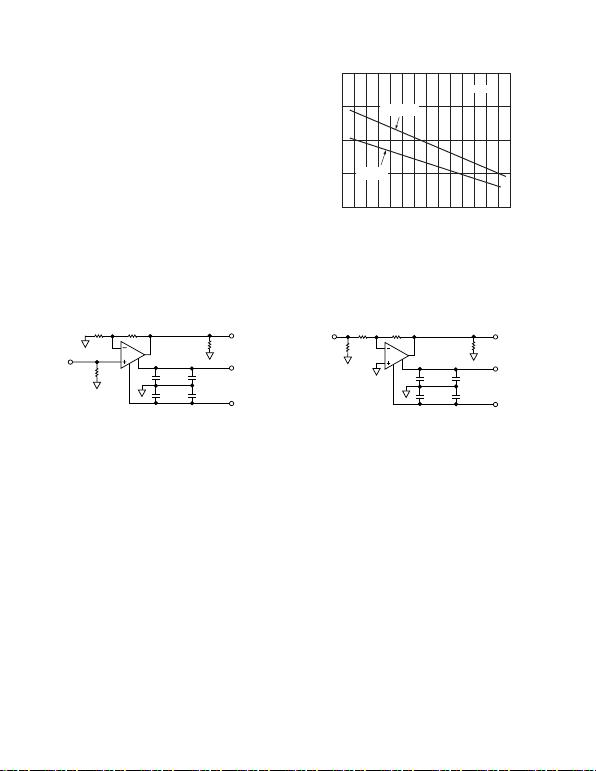REV. B–4–
MAXIMUM POWER DISSIPATION
The maximum power that can be safely dissipated by the AD8012
is limited by the associated rise in junction temperature. The maxi-
mum safe junction temperature for plastic encapsulated devices
is determined by the glass transition temperature of the plastic,
approximately +150°C. Temporarily exceeding this limit may
cause a shift in parametric performance due to a change in the
stresses exerted on the die by the package. Exceeding a junction
temperature of +175°C for an extended period can result in
device failure.
current capability. As a result, shorting the output to common
can cause the AD8012 to source or sink 500 mA. To ensure
proper operation, it is necessary to observe the maximum power
derating curves. Direct connection of the output to either power
supply rail can destroy the device.
AMBIENT TEMPERATURE – C
–50
0
TJ = 150C
2.0
1.5
1.0
MAXIMUM POWER DISSIPATION – W
PACKAGE
–40 –30 010203040506070 8090
MSOP
0.5
–20 –10
Figure 3. Plot of Maximum Power Dissipation vs.
0.1F
0.1F
10F
10F
RL
VIN
VOUT
750750
49.9+VS
–VS
+
+
Test Circuit 1. Gain = +2
0.1F
0.1F
10F
10F
RL
VIN VOUT
750750
53.6
+VS
–VS
+
+
Test Circuit 2. Gain = –1
Test Circuits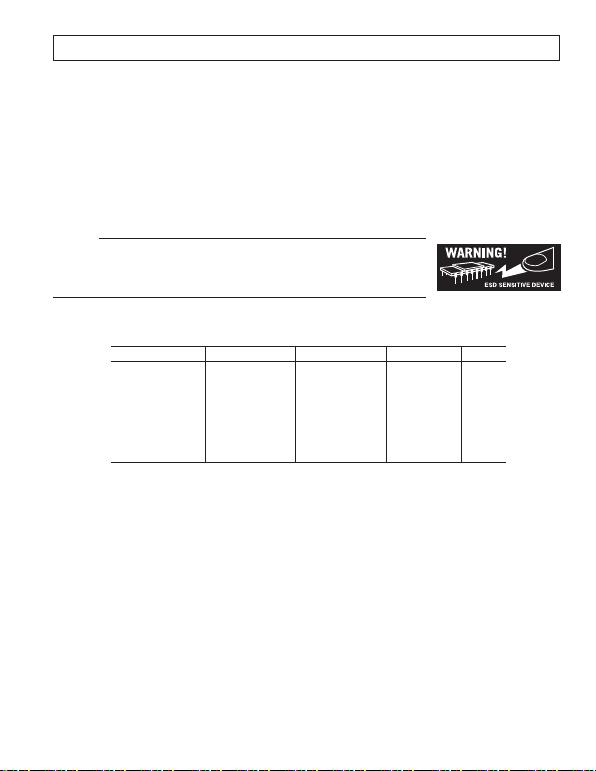REV. B
–5–
CAUTION
ESD (electrostatic discharge) sensitive device. Electrostatic charges as high as 4000 V readily
accumulate on the human body and test equipment and can discharge without detection. Although the
AD8012 features proprietary ESD protection circuitry, permanent damage may occur on devices
subjected to high energy electrostatic discharges. Therefore, proper ESD precautions are recommended
to avoid performance degradation or loss of functionality.
ABSOLUTE MAXIMUM RATINGS
1
Supply Voltage . . . . . . . . . . . . . . . . . . . . . . . . . . . . . . . . 12.6 V
Internal Power Dissipation
2
SOIC Package (R) . . . . . . . . . . . . . . . . . . . . . . . . . . . 0.8 W
MSOP Package (RM) . . . . . . . . . . . . . . . . . . . . . . . . 0.6 W
Input Voltage (Common Mode) . . . . . . . . . . . . . . . . . . . . ±V
S
Differential Input Voltage . . . . . . . . . . . . . . . . . . . . . . . ±2.5 V
Output Short-Circuit Duration
. . . . . . . . . . . . . . . . . .Observe Power Derating Curves
Storage Temperature Range RM, R . . . . . . 65°C to +125°C
Operating Temperature Range (A Grade) . . . 40°C to +85°C
Lead Temperature Range (Soldering 10 sec) . . . . . . . . . 300°C
NOTES
1
Stresses above those listed under Absolute Maximum Ratings may cause perma-
nent damage to the device. This is a stress rating only; functional operation of the
device at these or any other conditions above those indicated in the operational
section of this specification is not implied. Exposure to absolute maximum rating
conditions for extended periods may affect device reliability.
2
Specification is for device in free air at +25°C.
JA
= 155°C/W
JA
= 200°C/W
ORDERING GUIDE
Model Temperature Range Package Description Package Options Branding
AD8012AR-REEL 40°C to +85°C13 Tape and Reel R-8
AD8012AR-REEL7 40°C to +85°C7 Tape and Reel R-8
AD8012ARM-REEL 40°C to +85°C13 Tape and Reel RM-08 H6A
AD8012ARM-REEL7 40°C to +85°C7 Tape and Reel RM-08 H6A
AD8012ARMZ-REEL*40°C to +85°C13 Tape and Reel RM-08 H6A
AD8012ARMZ-REEL7*40°C to +85°C7 Tape and Reel RM-08 H6A
*Z = Pb-free product.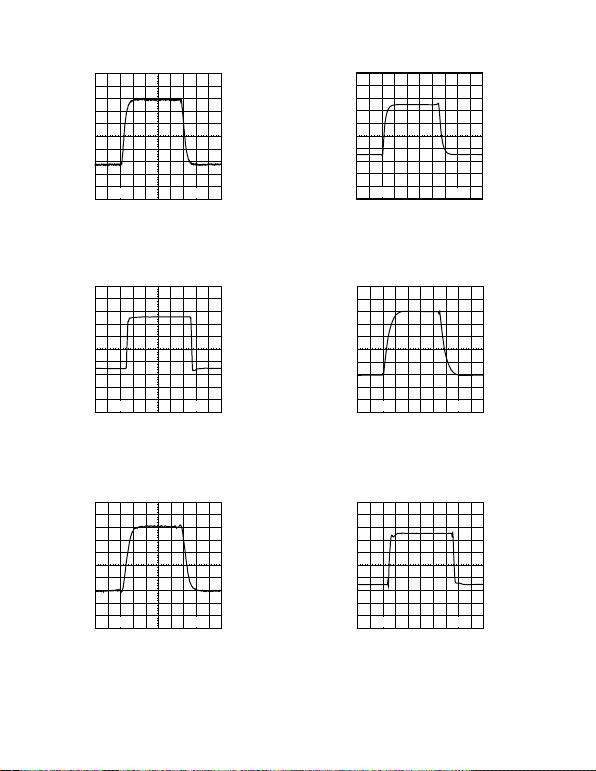REV. B–6–
20mV 5ns
TPC 1. 100 mV Step Response; G = +2, V
S
=
±
2.5 V or
±
5 V, R
L
= 1 k
*
1V 10ns
TPC 2. 4 V Step Response; G = +2, V
S
=
±
5 V, R
L
= 1 k
20mV 5ns
TPC 3. 100 mV Step Response; G = –1, V
S
=
±
2.5 V
or
±
5 V, R
L
= 1 k
*
1V 10ns
TPC 4. 4 V Step Response; G = –1, V
S
=
±
5 V, R
L
= 1 k
20mV 5ns
TPC 5. 100 mV Step Response; G = +2, V
S
=
±
2.5 V or
±
5 V, R
L
= 100
*
500mV 10ns
TPC 6. 2 V Step Response; G = +2, V
S
=
±
2.5 V, R
L
= 100
*V
S
= ±2.5 V operation is identical to V
S
= +5 V single-supply operation.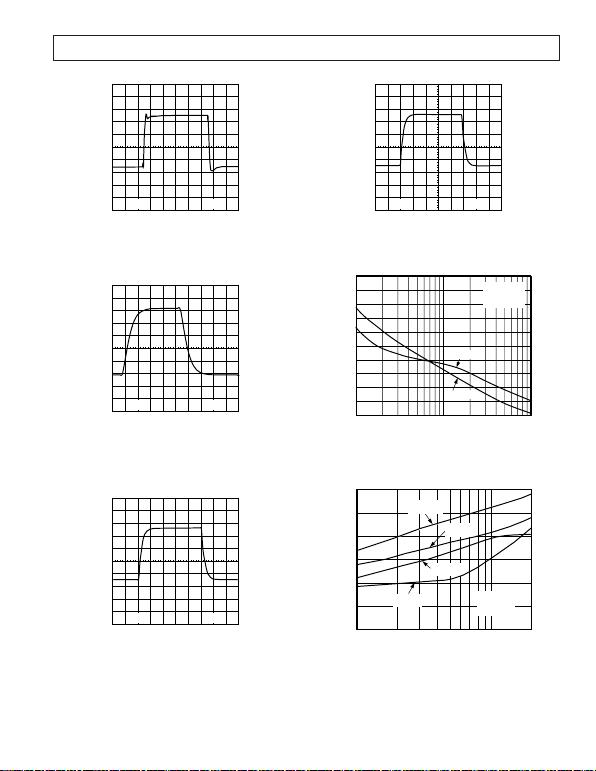REV. B
–7–
1V 10ns
TPC 7. 4 V Step Response; G = +2, V
S
=
±
5 V, R
L
= 100
20mV 5ns
TPC 8. 100 mV Step Response; G = –1, V
S
=
±
2.5 V
or
±
5 V, R
L
= 100
*
500mV 10ns
TPC 9. 2 V Step Response; G = –1, V
S
=
±
2.5 V, R
L
= 100
1V 10ns
TPC 10. 4 V Step Response; G = –1, V
S
=
±
5 V, R
L
= 100
R
L
–40
–9010 1k100
DISTORTION – dBc
–70
–80
–60
–50
G = +2
V
OUT
= 2V p-p
R
F
= 750
THIRD
SECOND
TPC 11. Distortion vs. Load Resistance; V
S
=
±
5 V,
Frequency = 500 kHz
THIRD
R
L
= 1k
FREQUENCY MHz
DISTORTION dBc
110 20
SECOND
R
L
= 1k
THIRD
R
L
= 100
SECOND
R
L
= 100
–40
–60
–80
–100
G = +2
V
OUT =
2V p-p
R
F
= 750
TPC 12. Distortion vs. Frequency; V
S
=
±
5 V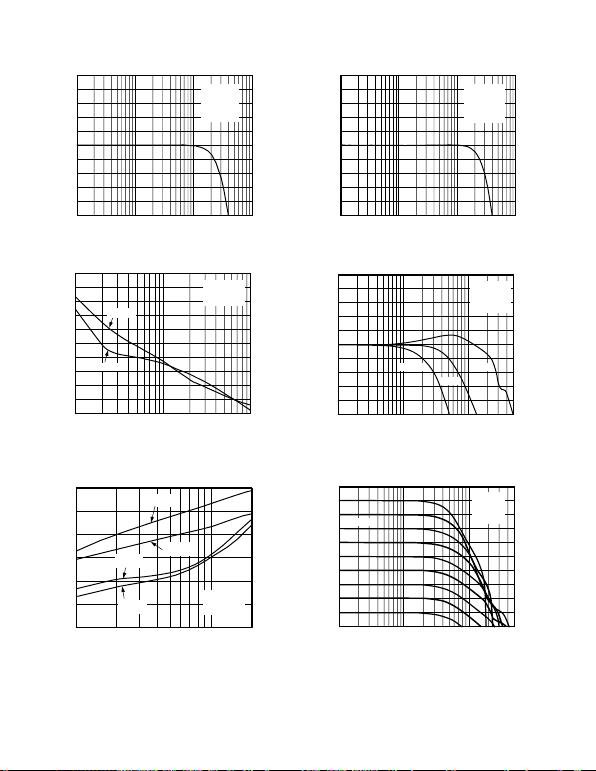REV. B–8–
FREQUENCY MHz
0.1 10 100
G = +2
VO = 0.3V p-p
RF = 750
RL = 100
VS = 5V
1
0.3
–0.3
0.2
–0.1
0.1
0
–0.2
–0.4
–0.5
0.4
0.5
NORMALIZED GAIN dB
TPC 13. Gain Flatness; V
S
=
±
5 V
RL
–40
–9010 1k100
DISTORTION – dBc
–70
–80
–60
–50
G = +2
VOUT = 2V p-p
RF = 750
SECOND
THIRD
TPC 14. Distortion vs. Load Resistance; V
S
= +5 V,
Frequency = 500 kHz
–40
–100
10 20
DISTORTION – dBc
–80
–60
G = +2
VOUT = 2V p-p
RF = 750
FREQUENCY – MHz
1
THIRD
RL = 1k
SECOND
RL = 1k
THIRD
RL = 100
SECOND
RL = 100
TPC 15. Distortion vs. Frequency; V
S
= +5 V
FREQUENCY MHz
0.1 10 100
G = +2
VO = 0.3V p-p
RF = 750
RL = 100
VS = +5V
1
0.3
–0.3
0.2
–0.1
0.1
0
–0.2
–0.4
–0.5
0.4
0.5
NORMALIZED GAIN dB
TPC 16. Gain Flatness; V
S
= +5 V
FREQUENCY MHz
3
–3
100 500
2
–1
VO = 0.3V p-p
RF = 750
RL = 100
VS = 5V
1
0
–2
–4
4
5
G = +10
G = +2
G = +1
–5
10
1
NORMALIZED GAIN dB
TPC 17. Frequency Response; V
S
=
±
5 V
FREQUENCY MHz
3
–15
100 500
0
–9
G = +2
RF = 750
RL = 100
VS = 5V
–3
–6
–12
–18
6
9
–21
10
1
OUTPUT VOLTAGE dBV
1V RMS
TPC 18. Output Voltage vs. Frequency; V
S
=
±
5 V,
G = +2, R
L
= 100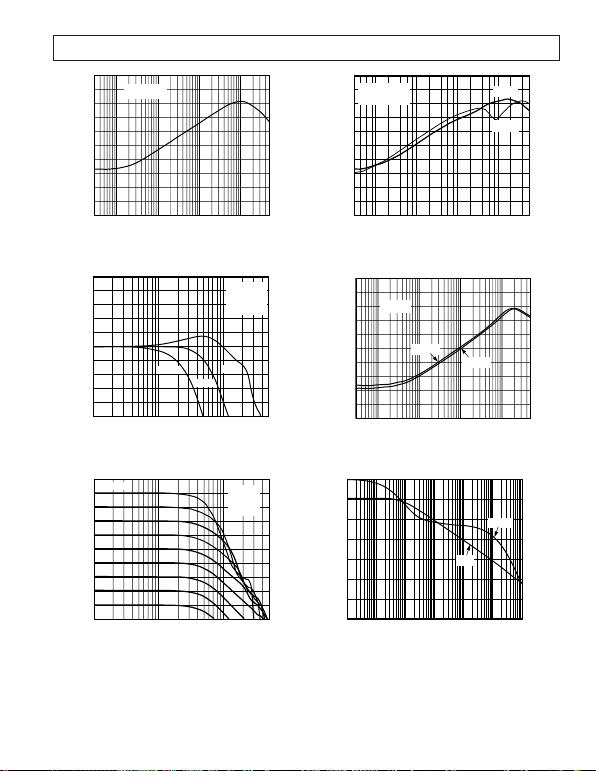REV. B
–9–
FREQUENCY – MHz
–20
–80
100 500
–30
–60
VIN = 0.2V p-p
VS = 5V, +5V
–40
–50
–70
–90
–10
0
10.03 0.1 10
CMRR dB
–100
TPC 19. CMRR vs. Frequency; V
S
=
±
5 V, +5 V
FREQUENCY – MHz
NORMALIZED GAIN dB
3
–3
100 500
2
–1
V
O
= 0.3V p-p
R
F
= 750
R
L
= 100
V
S
= +5V
1
0
–2
–4
4
5
G = +10
G = +2
G = +1
–5
10
1
TPC 20. Frequency Response; V
S
= +5 V
FREQUENCY MHz
OUTPUT VOLTAGE dBV
–3
–21
100 500
–6
–15
G = +2
RF = 750
RL = 100
VS = +5V
–9
–12
–18
–24
0
3
–27
10
1
1VRMS
TPC 21. Output Voltage vs. Frequency; V
S
= +5 V,
G = +2, R
L
= 100
0
–10
–20
–30
–40
–60
–70
–80
100k 1M 10M 100M 500M
FREQUENCY – Hz
–50
–90
–100
PSRR – dB
V
S
= +5V OR 5V
G = +2
R
F
= 750
–PSRR
+PSRR
TPC 22. PSRR vs. Frequency; V
S
=
±
5 V, +5 V
FREQUENCY – MHz
OUTPUT RESISTANCE
100
0.1
100 500
1
10
1k
0.01
10.03 0.1 10
VS = 5V
VS = +5V
G = +2
RF = 750
TPC 23. Output Resistance vs. Frequency
135
115
95
75
55
15
–5
FREQUENCY Hz
35
TZ dB
0
PHASE – Degrees
–40
–80
–120
–160
–200
–240
–280
TZ(s)
PHASE
1k 10k 100k 1M 10M 100M 1G
TPC 24. Open-Loop Transimpedance and
Phase vs. Frequency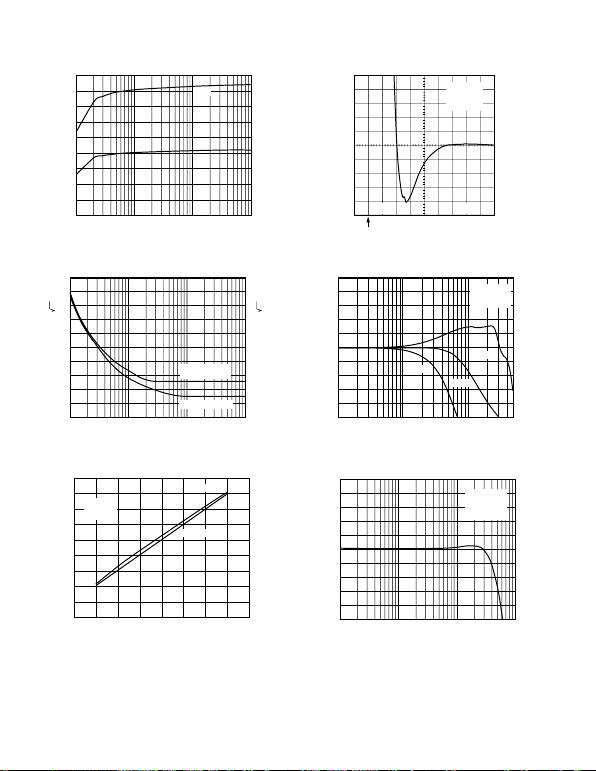REV. B–10–
10 1k 10k100
7
1
6
3
5
4
2
0
8
9
SWING – V p-p
+5V
5V
TPC 25. Output Swing vs. Load
FREQUENCY – Hz
100 10k1k
3.6
2.4
3.4
2.8
3.2
3.0
2.6
2.2
2.0
3.8
4.0
CURRENT NOISE
+IN/–IN
VOLTAGE NOISE
26
14
24
18
22
20
16
12
10
28
30
INPUT CURRENT NOISE – pA/ Hz
100k
INPUT VOLTAGE NOISE – nV/ Hz
TPC 26. Noise vs. Frequency
9
8
7
6
5
4
3
2
3 4 5 6 7 8 9 10 11
1
0
f = 5MHz
G = 2
RF = 750
RL = 100
RL = 1k
TOTAL SUPPLY VOLTAGE V
PEAK-TO-PEAK OUTPUT AT 5MHz (1% THD) V
TPC 27. Output Swing vs. Supply
0.1% 5ns
G = +2
RF = 750
RL = 100
2V STEP
t = 0
OUTPUT VOLTAGE ERROR – 0.1%/DIV
TPC 28. Settling Time, V
S
=
±
5 V
FREQUENCY – MHz
3
–3
100 500
2
–1
V
O
= 0.3V p-p
R
F
= 750
R
L
= 1k
1
0
–2
–4
4
5
G = +10
G = +2
G = +1
–5
10
1
NORMALIZED GAIN dB
TPC 29. Frequency Response; V
S
=
±
5 V
0.3
–0.3
0.2
–0.1
VO = 0.3V p-p
G = +2
RF = 750
RL = 1k
0.1
0
–0.2
–0.4
0.4
0.5
–0.5
FREQUENCY – MHz
0.1 101 100
NORMALIZED GAIN – dB
TPC 30. Gain Flatness; V
S
=
±
5 V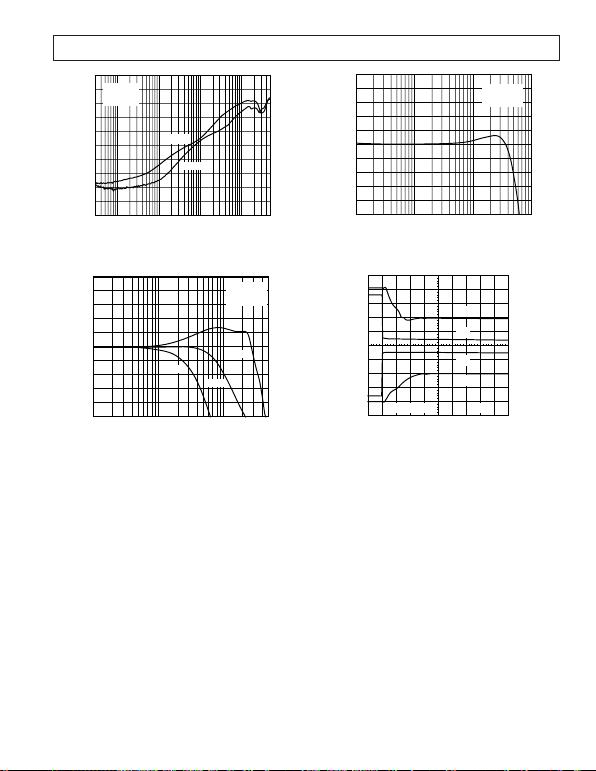REV. B
–11–
FREQUENCY – MHz
INPUT REFERRED ERROR – dB
–40
–100
100 500
–50
–80
–60
–70
–90
–110
–30
–20
–120
10.03 0.1 10
SIDE 1
SIDE 2
DRIVER
VO = 2V p-p
RL = 100
TPC 31. Crosstalk vs. Frequency
FREQUENCY – MHz
3
–3
100 500
2
–1
V
O
= 0.3V p-p
R
F
= 750
R
L
= 1k
1
0
–2
–4
4
5
G = +10
G = +2
G = +1
–5
10
1
NORMALIZED GAIN dB
TPC 32. Frequency Response; V
S
= +5 V
NORMALIZED GAIN – dB
0.3
–0.3
0.2
–0.1
VO = 0.3V p-p
RF = 750
RL = 1k
0.1
0
–0.2
–0.4
0.4
0.5
–0.5
FREQUENCY – MHz
0.1 101 100
TPC 33. Gain Flatness; V
S
= +5 V
V
OUT
, 2V/DIV 20ns
+3V
0V
0V
V
OUT
V
OUT
V
IN
V
IN
0V
0V
–3V
TPC 34. Overdrive Recovery; V
S
=
±
5 V, G = +2,
R
F
= 750
, R
L
= 100
, V
IN
= 3 V p-p (T = 1
µ
s)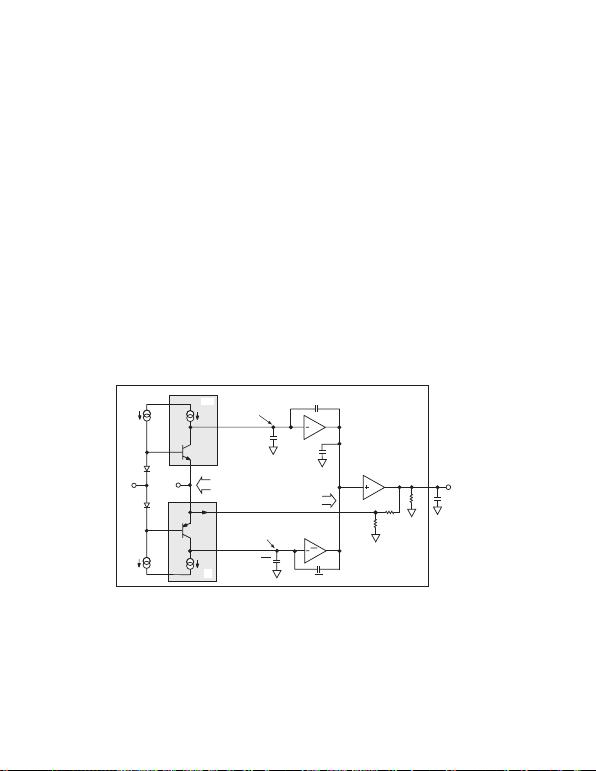REV. B–12–
THEORY OF OPERATION
The AD8012 is a dual, high speed CF amplifier that attains new
levels of bandwidth (BW), power, distortion, and signal swing
capability. Its wide dynamic performance (including noise) is
the result of both a new complementary high speed bipolar
process and a new and unique architectural design. The AD8012
uses a two-gain stage complementary design approach versus
the traditional single-stage complementary mirror structure
sometimes referred to as the Nelson amplifier. Though twin
stages have been tried before, they typically consumed high
power since they were of a folded cascade design, similar to that
of the AD9617. This design allows for the standing or quiescent
current to add to the high signal or slew current-induced stages.
In the time domain, the large signal output rise/fall time and
slew rate is typically controlled by the small signal BW of the
amplifier and the input signal step amplitude, respectively, and
not the dc quiescent current of the gain stages (with the excep-
tion of input level shift diodes Q1/Q2). Using two stages versus
one also allows for a higher overall gain bandwidth product
(GBWP) for the same power, resulting in lower signal distortion
second-gain stage also isolates (divides down) A3s input
reflected load drive and the nonlinearities created, resulting in
relatively lower distortion and higher open-loop gain.
Overall, when high external load drive and low ac distortion is a
requirement, a twin-gain stage integrating amplifier like the
AD8012 will provide excellent results for lower power over the
because the AD8012 is a CF amplifier, closed-loop BW variations
versus external gain variations (varying RN) will be much lower
compared to a VF op amp, where the BW varies inversely with
gain. Another key attribute of this amplifier is its ability to run on
a single 5 V supply partially because of its wide common-mode
input and output voltage range capability. For 5 V supply
operation, the device consumes half the quiescent power (vs.
10 V supply) with little degradation in its ac and dc perfor-
mance characteristics. See data sheet comparisons.
DC GAIN CHARACTERISTICS
Gain stages A1/A1B and A2/A2B combined provide negative
feedforward transresistance gain as shown in Figure 4. Stage A3
is a unity-gain buffer that provides external load isolation to A2.
Each stage uses a symmetrical complementary design (A3 is also
complementary though not explicitly shown). This is done to
reduce both second-order signal distortion and overall quiescent
power as previously described. In the quasi dc to low frequency
region, the closed-loop gain relationship can be approximated as:
These basic relationships are common to all traditional opera-
tional amplifiers.
VP
Q1
Q2
IPP IPN
INP IPN
VN
A1
A1
ZI
IQ1
Q3
Q4
IE
CP1
CP1
Z2
A2
CL
RN
ICQ – IO
RF
VO
CD
ICQ + IO
IQ1
A2
CP2
Z1 = R1 || C1
Z1
CD
A3
RL
Z1
–VI
–VI
IR – IFC
IR + IFC
+ Ð
VOI
Figure 4. Simplified Block Diagram
G= +R R
G=–R R
FN
FN
1/
/
noninverting operation
inverting operation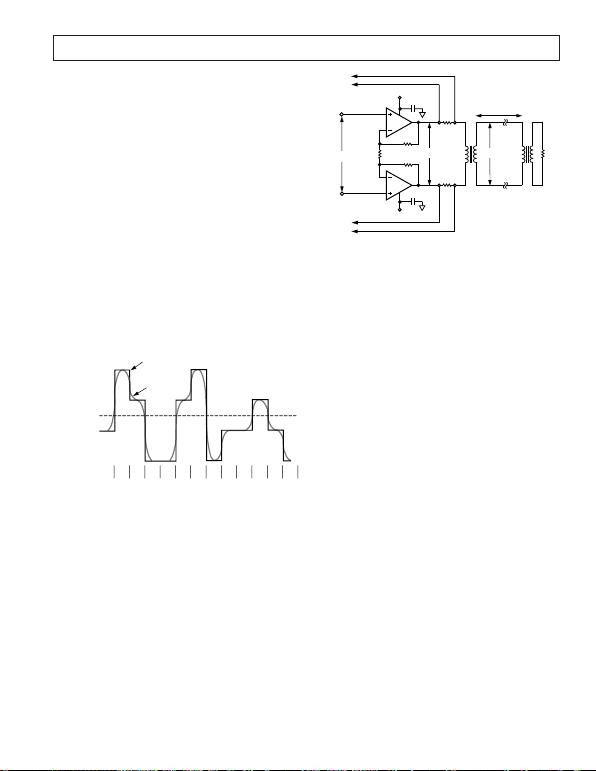REV. B
–13–
APPLICATIONS
Line Driving for HDSL
High bitrate digital subscriber line (HDSL) is becoming
popular as a means of providing full duplex data communication at
rates up to 1.544 MBPS or 2.048 MBPS over moderate distances
via conventional telephone twisted pair wires. Traditional T1
(E1 in Europe) requires repeaters every 3,000 feet to 6,000 feet
to boost the signal strength and allow transmission over distances
of up to 12,000 feet. In order to achieve repeaterless transmission
over this distance, an HDSL modem requires a transmitted
power level of 13.5 dBm (assuming a line impedance of 135 ).
HDSL uses the two binary/one quaternary line code (2B1Q).
A sample 2B1Q waveform is shown in Figure 5. The digital bit
stream is broken up into groups of two bits. Four analog volt-
ages (called quaternary symbols) are used to represent the four
possible combinations of two bits. These symbols are assigned
the arbitrary names +3, +1, 1, and 3. The corresponding
voltage levels are produced by a DAC that is usually part of an
analog front end circuit (AFEC). Before being applied to the
line, the DAC output is low-pass filtered and acquires the sinu-
soidal form shown in Figure 5. Finally, the filtered signal is
applied to the line driver. The line voltages that correspond to
the quaternary symbols +3, +1, 1, and 3 are 2.64 V, 0.88 V,
0.88 V, and 2.64 V. This gives a peak-to-peak line voltage of
5.28 V.
VOLTAGE
+3 2.64V
+1 0.88V
–1 –0.88V
–3 –2.64V
SYMBOL
NAME DAC
OUTPUT
FILTERED
OUTPUT
TO LINE
DRIVER
–1
01
+3
10
+1
11
–3
00
–3
00
+1
11
+3
10
–3
00
–1
01
–1
01
+1
11
–1
01
–3
00
Figure 5. Time Domain Representation of an HDSL Signal
Many of the elements of a classic differential line driver are
shown in the HDSL line driver in Figure 6. A 6 V peak-to-peak
differential signal is applied to the input. The differential gain of
the amplifier (1+2 R
F
/R
G
) is set to +2, so the resulting differen-
tial output signal is 12 V p-p.
As is normal in telephony applications, a transformer galvani-
cally isolates the differential amplifier from the line. In this case,
a 1:1 turns ratio is used. In order to correctly terminate the line,
it is necessary to set the output impedance of the amplifier to be
equal to the impedance of the line being driven (135 in this
case). Because the transformer has a turns ratio of 1:1, the
impedance reflected from the line is equal to the line impedance
of 135 (R
REFL
= R
LINE
/Turns Ratio
2
). As a result, two 66.5
resistors correctly terminate the line.
6V p-p 12V p-p
1:1
+5V
–5V
RF
750
RF
750
RG
1.5k
1/2
1/2
0.1F
0.1F
66.5
66.5
6V p-p
1:1
135
TO
CIRCUITRY
TO
CIRCUITRY
GAIN = +2
UP TO
12,000 FEET
+
Figure 6. Differential for HDSL Applications
The immediate effect of back-termination is that the signal from
the amplifier is halved before being applied to the line. This
doubles the power the amplifier must deliver. However, the
back-termination resistors also play an important second role.
Full-duplex data transmission systems like HDSL simulta-
neously transmit data in both directions. As a result, the signal
on the line and across the back termination resistors is the
composite of the transmitted and received signal. The termina-
tion resistors are used to tap off this signal and feed it to the
being transmitted, the transmitted data can be subtracted from
the digitized composite signal to reveal the received data.
Driving a line with a differential signal offers a number of
advantages compared to a single-ended drive. Because the two
outputs are always 180 degrees out of phase relative to one
another, the differential signal output is double the output
amplitude of either of the op amps. As a result, the differential
amplifier can have a peak-to-peak swing of 16 V (each op amp
can swing to ±4 V), even though the power supply is ±5 V.
In addition, even-order harmonics (second, fourth, sixth, and
so on.) of the two single-ended outputs tend to cancel out one
another, so the total harmonic distortion (quadratic sum of all
harmonics) decreases compared to the single-ended case, even
as the signal amplitude is doubled. This is particularly advan-
tageous in the case of the second harmonic. Because it is very
close to the fundamental, filtering becomes difficult. In this
application, the THD is dominated by the third harmonic,
which is 65 dB below the carrier (i.e., spurious-free dynamic
range = 65 dBc).
Differential line driving also helps to preserve the integrity of the
transmitted signal in the presence of electromagnetic interfer-
ence (EMI). EMI tends to induce itself equally onto both the
positive and negative signal lines. As a result, a receiver with
good common-mode rejection will amplify the original signal
while rejecting induced (common-mode) EMI.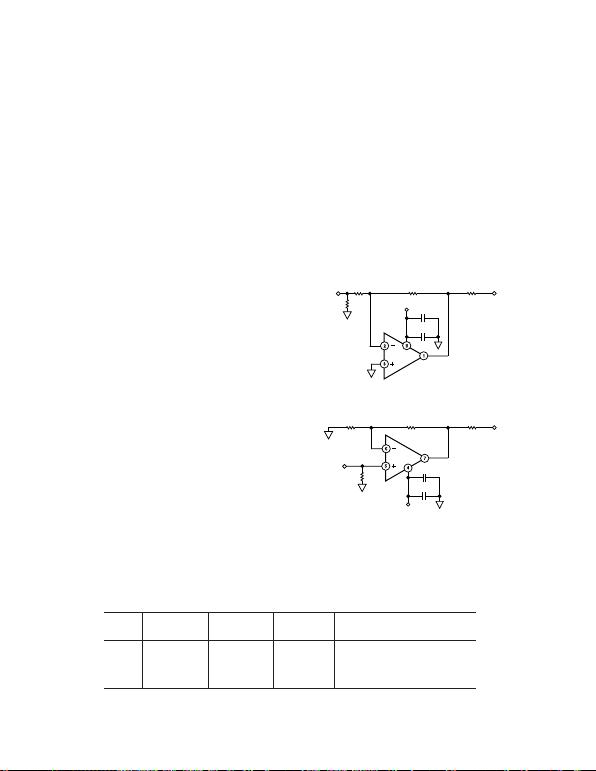REV. B–14–
Choosing the Appropriate Turns Ratio for the Transformer
Increasing the peak-to-peak output signal of the amplifier in the
previous example and adding a variation in the turns ratio of the
transformer can yield further enhancements to the circuit. The
±3.9 V before clipping occurs. This increases the peak-to-peak
output of the differential amplifier to 15.6 V. Because the signal
applied to the primary winding is now bigger, the transformer
turns ratio of 1:1 can be replaced with a (step-down) turns ratio
of about 1.3:1 (from amplifier to line). This steps the 7.8 V
peak-to-peak primary voltage down to 6 V. This is the same
secondary voltage of the earlier examples, so the resulting power
delivered to the line is the same.
The received signal, which is small relative to the transmitted
signal, will, however, be stepped up by a factor of 1.3. Amplifying
the received signal in this manner enhances its signal-to-noise
ratio and is useful when the received signal is small compared to
the to-be-transmitted signal.
The impedance reflected from the 135 line now becomes
228 (1.3
2
135 ). With a correctly terminated line, the
amplifier must now drive a total load of 456 (114 + 114
+ 228 ), considerably more than the original 270 load. This
reduces the drive current from the op amps by about 40%.
More significant, however, is the reduction in dynamic power
consumptionthat is, the power the amplifier must consume in
order to deliver the load power. Increasing the output signal so
that it is as close as possible to the power rails minimizes the
power consumed in the amplifier.
There is, however, a price to pay in terms of increased signal
distortion. Increasing the output signal of each op amp from the
original ±3 V to ±3.9 V reduces the spurious-free dynamic
range (SFDR) from 65 dB to 50 dB (measured at 500 kHz),
even though the overall load impedance has increased from
270 to 456 .
LAYOUT CONSIDERATIONS
The specified high speed performance of the AD8012 requires
careful attention to board layout and component selection.
Table I shows recommended component values for the AD8012
and Figures 813 show recommended layouts for the 8-lead
SOIC and MSOP packages for a positive gain. Proper RF
design techniques and low parasitic component selections
are mandatory.
The PCB should have a ground plane covering all unused
portions of the component side of the board to provide a low
impedance ground path. The ground plane should be removed
from the area near the input pins to reduce stray capacitance.
Chip capacitors should be used for supply bypassing (see Figure 7).
One end should be connected to the ground plane and the other
within 1/8 inch of each power pin. An additional (4.7 µF to 10 µF)
tantalum electrolytic capacitor should be connected in parallel.
The feedback resistor should be located close to the inverting
input pin in order to keep the stray capacitance at this node to
a minimum. Capacitance greater than 1.5 pF at the inverting
input will significantly affect high speed performance when
operating at low noninverting gains.
Stripline design techniques should be used for long signal traces
(greater than about 1 inch). They should be designed with the
proper system characteristic impedance and be properly termi-
nated at each end.
0.1F
INVERTING CONFIGURATION
VOUT
RF
10F
NONINVERTING CONFIGURATION
VOUT
RGRF
RT
0.1F
10F
RT
VIN
RG
VIN
*RO CHOSEN FOR CHARACTERISTIC IMPEDANCE.
+VS
+
+
–VS
RO*
RO*
*RO CHOSEN FOR CHARACTERISTIC IMPEDANCE.
Figure 7. Inverting and Noninverting Configurations
Table I. Typical Bandwidth vs. Gain Setting Resistors
Small Signal 3 dB BW (MHz),
Gain R
F
R
G
R
T
V
S
= 5 V, R
L
= 1 k
1750 750 53.6 110
+1 750 49.9 350
+2 750 750 49.9 150
+10 750 82.5 49.9 40
R
T
chosen for 50 characteristic input impedance.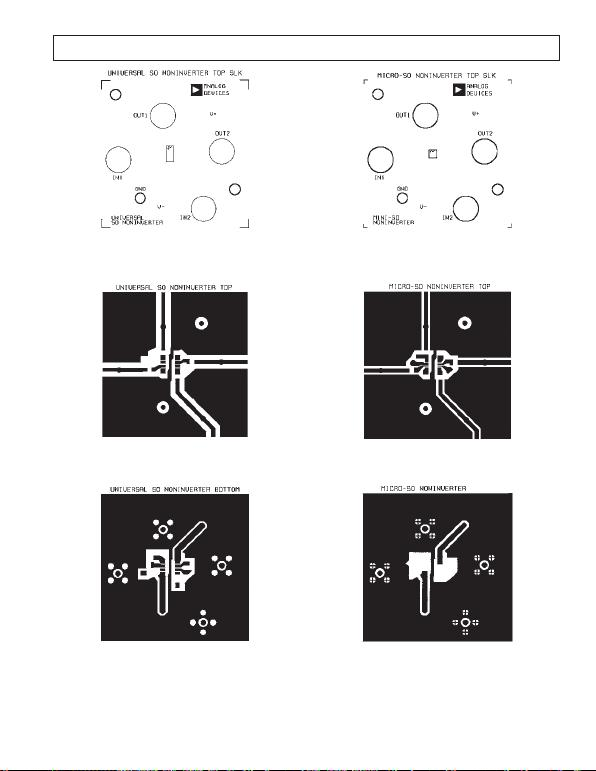REV. B
–15–
Figure 8. Universal SOIC Noninverter Top Silkscreen
Figure 9. Universal SOIC Noninverter Top
Figure 10. Universal SOIC Noninverter Bottom
Figure 11. Universal MSOP Noninverter Top
Silkscreen
Figure 12. Universal MSOP Noninverter Top
Figure 13. Universal MSOP Noninverter Bottom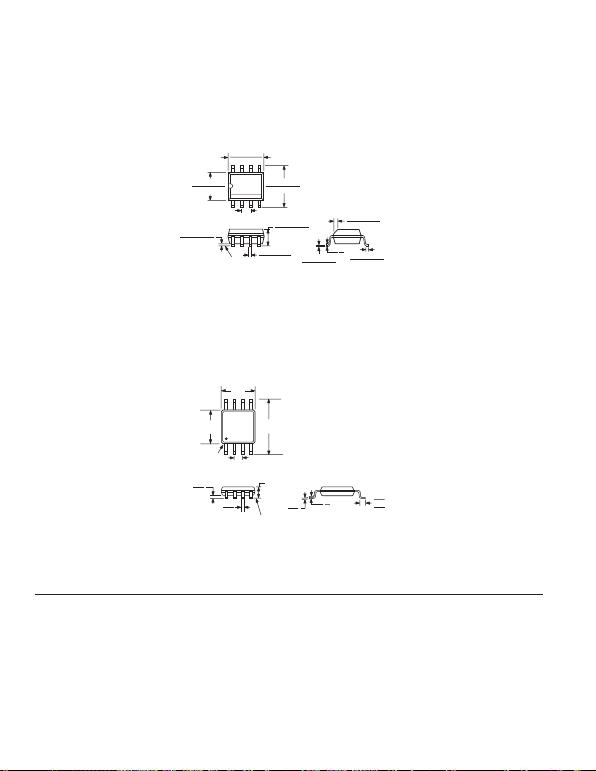REV. B
C01049–0–12/03(B)
–16–
OUTLINE DIMENSIONS
8-Lead Standard Small Outline Package [SOIC]
Narrow Body
(R-8)
Dimensions shown in millimeters and (inches)
0.25 (0.0098)
0.17 (0.0067)
1.27 (0.0500)
0.40 (0.0157)
0.50 (0.0196)
0.25 (0.0099) 45
8
0
1.75 (0.0688)
1.35 (0.0532)
SEATING
PLANE
0.25 (0.0098)
0.10 (0.0040)
85
41
5.00 (0.1968)
4.80 (0.1890)
4.00 (0.1574)
3.80 (0.1497)
1.27 (0.0500)
BSC
6.20 (0.2440)
5.80 (0.2284)
0.51 (0.0201)
0.31 (0.0122)
COPLANARITY
0.10
CONTROLLING DIMENSIONS ARE IN MILLIMETERS; INCH DIMENSIONS
(IN PARENTHESES) ARE ROUNDED-OFF MILLIMETER EQUIVALENTS FOR
REFERENCE ONLY AND ARE NOT APPROPRIATE FOR USE IN DESIGN
COMPLIANT TO JEDEC STANDARDS MS-012AA
8-Lead Mini Small Outline Package [MSOP]
(RM-8)
Dimensions shown in millimeters
0.80
0.60
0.40
8
0
85
4
1
4.90
BSC
PIN 1
0.65 BSC
3.00
BSC
SEATING
PLANE
0.15
0.00
0.38
0.22
1.10 MAX
3.00
BSC
COPLANARITY
0.10
0.23
0.08
COMPLIANT TO JEDEC STANDARDS MO-187AA
Revision History
Location Page
12/03Data Sheet changed from REV. A to REV. B.
Renumbered figures and TPCs . . . . . . . . . . . . . . . . . . . . . . . . . . . . . . . . . . . . . . . . . . . . . . . . . . . . . . . . . . . . . . . . . . . . . . .Universal
Updated ORDERING GUIDE . . . . . . . . . . . . . . . . . . . . . . . . . . . . . . . . . . . . . . . . . . . . . . . . . . . . . . . . . . . . . . . . . . . . . . . . . . . . . 5
Updated OUTLINE DIMENSIONS . . . . . . . . . . . . . . . . . . . . . . . . . . . . . . . . . . . . . . . . . . . . . . . . . . . . . . . . . . . . . . . . . . . . . . . 16